Courses

O P Tandon Test: Some Basic Principles

30 Questions MCQ Test Chemistry for JEE | O P Tandon Test: Some Basic Principles

Description
Attempt O P Tandon Test: Some Basic Principles | 30 questions in 45 minutes | Mock test for Class 11 preparation | Free important questions MCQ to study Chemistry for JEE for Class 11 Exam | Download free PDF with solutions
QUESTION: 1

The compounds C2H5OC2H5 and CH3OCH2CH2CH3 are:

Solution:
• Chain isomerism occurs due to the possibility of branching in carbon chains.
• Geometrical isomerism or configurational isomerism or cis-trans isomerism arises when the functional group are present on same side of carbon or are on opposite side of the carbon chain.
• Metamerism arises when organic compounds have the same molecular formula but different alkyl groups on either side of the functional group.
• In C2H5OC2H5 and CH3OCH2CH2CH3  , we can see that in diethyl ether we have 2 carbon atoms on the left side of oxygen but in methyl propyl ether we have one carbon atom on the left of oxygen. On the right hand side of oxygen, we have 2 carbon atoms but in methyl propyl ether, we have 3 carbon atoms.

So, we can say that above molecules are metamers. Therefore, option C is correct.

QUESTION: 2

C7H7Cl shows how many benzenoid aromatic isomers?

Solution:

4 aromatic isomers are possible.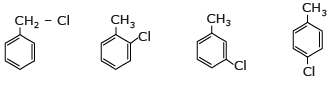QUESTION: 3

How many minimum no. of C-atoms are required for position & geometrical isomerism in alkene?

Solution:

For positional isomerism in alkene 4C-atom required →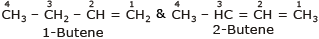For geometrical isomerism in alkene also 4c–atom required →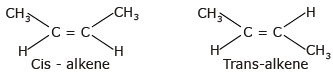QUESTION: 4

How many structural formula are possible when one of the hydrogen is replaced by a chlorine atom in anthracene?

Solution:

There are 3 different positions of hydrogen. Hence, 3 structural isomers are possible.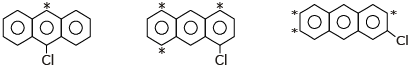QUESTION: 5

The principle involved in paper chromatography is:

Solution:
• Partition chromatography because the substances are partitioned or distributed between liquid phases.
• The two phases are water held in pores of the filter paper and the other phase is a mobile phase that passes through the paper.
QUESTION: 6

The number of cis-trans isomer possible for the following compound: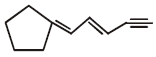Solution:

Cis-trans Isomers:- It is under the class of geometric isomer,

• It exists in alkenes (organic molecules which have double bonds).
• When two similar or higher priority groups are attached to the carbon on the same side, it is termed as cis isomer and when it is attached to the opposite side it is called a trans isomer.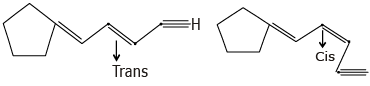QUESTION: 7

The number of isomers of dibromoderivative of an alkene (molear mass 186 g mol-1) is

Solution: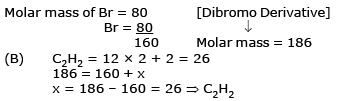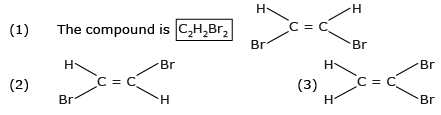QUESTION: 8

Increasing order of stability among the three main conformation (i.e. eclipse, anti, gauche) of ethylene glycol is:

Solution: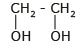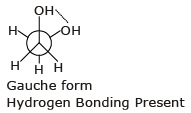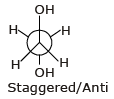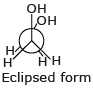Stability order ⇒ Gauche > Anti > Eclipse

QUESTION: 9

How many primary amines are possible for the formula C4H11N?

Solution:

Four primary amines are possible with the molecular formula C4H11N.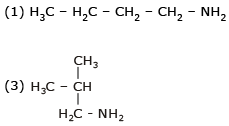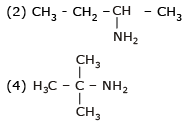QUESTION: 10

In which of the following compounds the carbon marked with asterisk is expected to have greatest positive charge?

Solution:

In CH3 - CH- Cl, the carbon marked with asterisk has the greatest positive charge due to high electronegativity of Cl.

QUESTION: 11

How many planes (pos) are presents in Anthracene?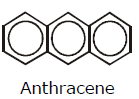Solution: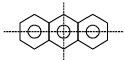(1) Vertical plane (2) Horizontal plane (3) Molecular Plane

QUESTION: 12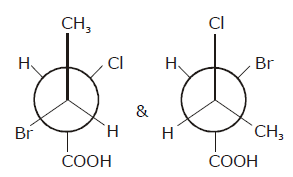Solution: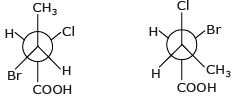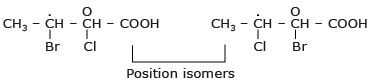QUESTION: 13

Geometrical isomers can be:

Solution:

Geometrical Isomers can be Diastereomers or Enantiomers.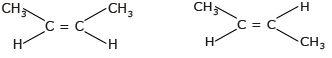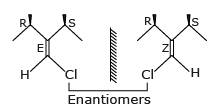Because geometrical isomers are around restricted rotation which can not be rotate or interconvertable.

QUESTION: 14

Phenol and benzyl alcohol are:

Solution:
• Phenol is C6​H5​(OH) while benzyl alcohol is C6H5​(CH2​OH).
• Here there is a difference of −HC2​ between their molecular formula.
• According to the definition of homologous series, the adjacent members of the series differ by −CH2​ group.
• Thus Phenol and benzyl alcohol are homologous of each other.
QUESTION: 15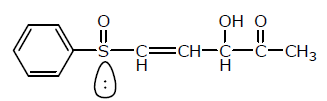Number of chiral centers are:

Solution: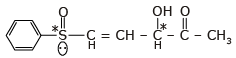Number of chiral centers = 2

QUESTION: 16

Which of the follownig compounds is (S)-4-chloro-1-methylcyclohexene ?

Solution:

(B) & (C) compounds are R-configuration.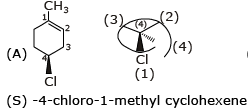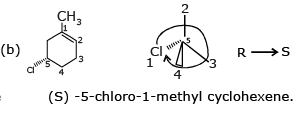QUESTION: 17

Which of the following compounds has two stereogenic centers (asymmetric carbons) ?

Solution: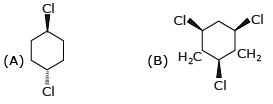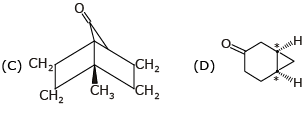QUESTION: 18

Which of the following structures represents a chiral compound ?

Solution: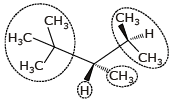All four substituent are different so it is chiral compound.

QUESTION: 19

Examine the compound on the right. How many stereoisomers having this constitution are possible ?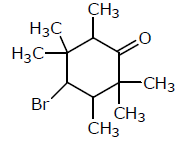Solution: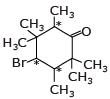Asymmetric = 23 = 8

QUESTION: 20

Which of the following heptanols are chiral 1-heptanol, 2-heptanol, 3-heptanol, 4-heptanol.

Solution: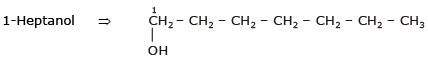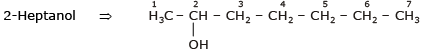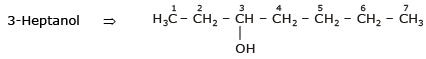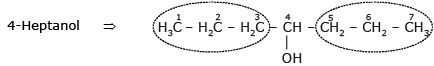1-Heptanol & 4-Heptanol are not chiral
2-Heptanol & 3-Heptanol are chiral compound.

QUESTION: 21

Which of the following will exhibit geometrical isomerism ?

Solution: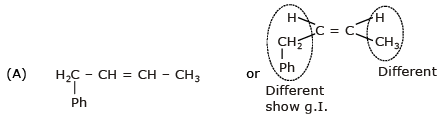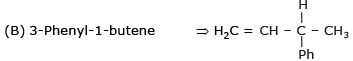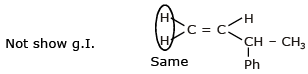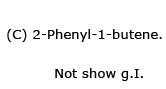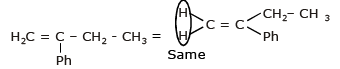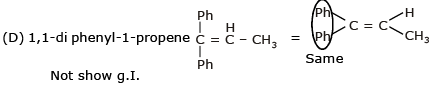QUESTION: 22

(A)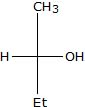(B)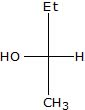Relation between given pair is

Solution: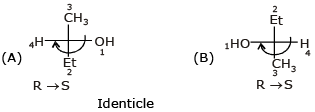QUESTION: 23

Following eclipsed form of propane is repeated after rotation of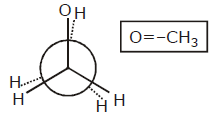Solution: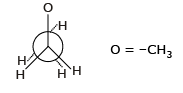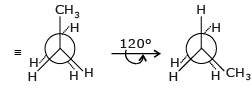QUESTION: 24

Stereoisomers differ from each other in what respect ?

Solution:

Stereoisomer differ from each other in configuration.

QUESTION: 25

The number of isomers of C5H10 is

Solution: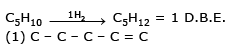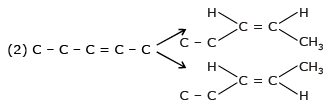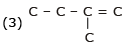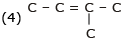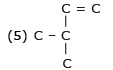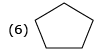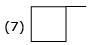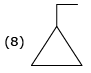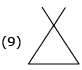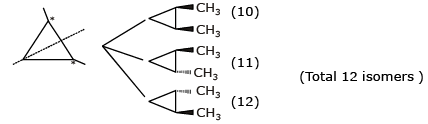QUESTION: 26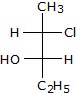The compound with the above configuration is called.

Solution: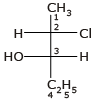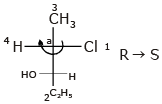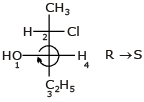(2s, 3s) -2-chloro-3-Hydroxy Pentane

QUESTION: 27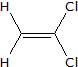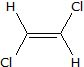The above compounds differ in

Solution: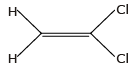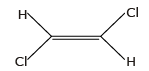The above compounds are different in structure.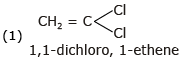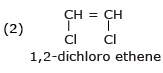QUESTION: 28

Which conformer of cyclohexane is chiral?

Solution:

Twisted Boat form is chiral & optically active.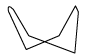QUESTION: 29

Minimum C atoms required for a compound to show geometrical isomerism:

Solution:

The smallest alkene showing geometrical isomerism is but−2−ene (4−C atoms).

QUESTION: 30

Which among the following does not exhibit geometric isomerism?

Solution:

Alkenes like 1-hexene when flipped from top to bottom they have identical structures and also they have C=CH2 unit which does not exist as cis-trans isomers.Use Code STAYHOME200 and get INR 200 additional OFF Use Coupon Code

Track your progress, build streaks, highlight & save important lessons and more!

Similar ContentRelated tests